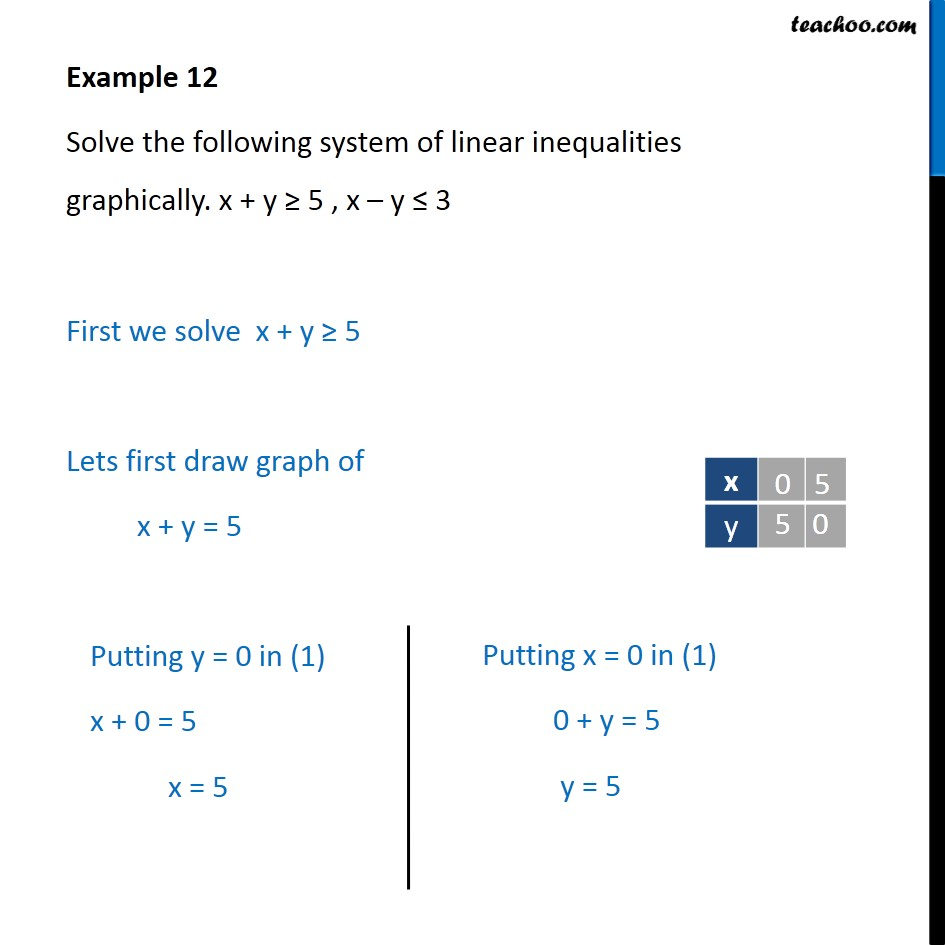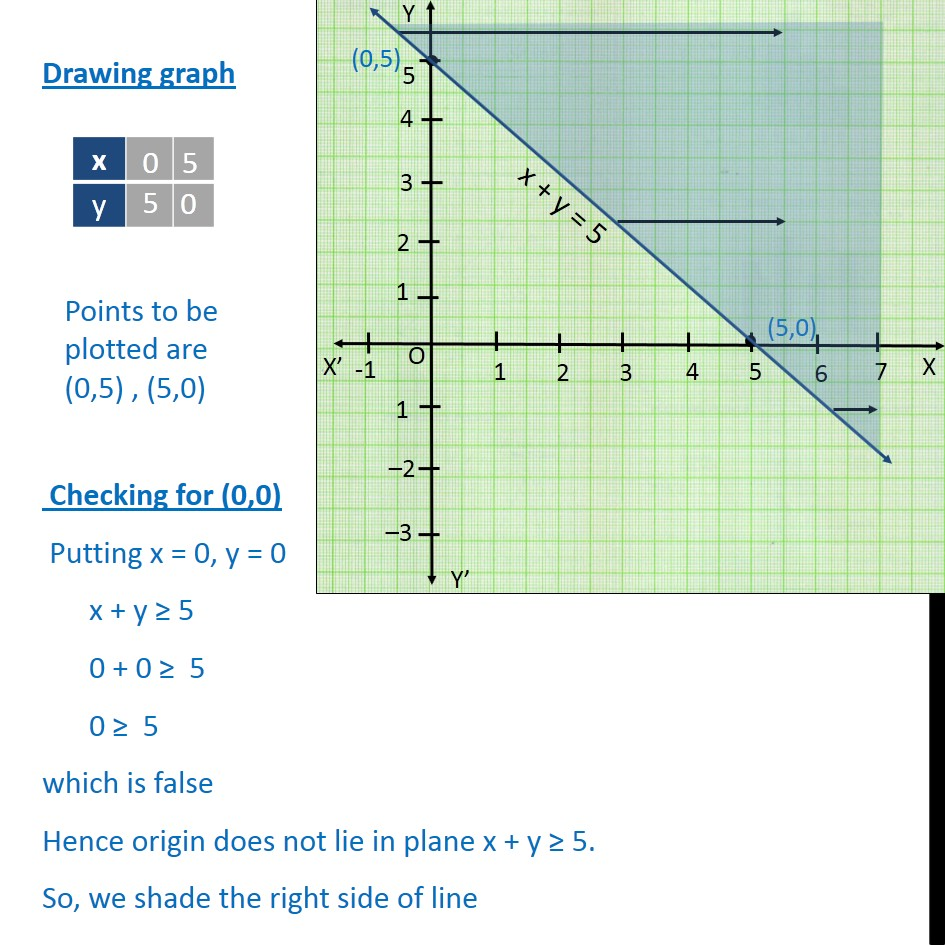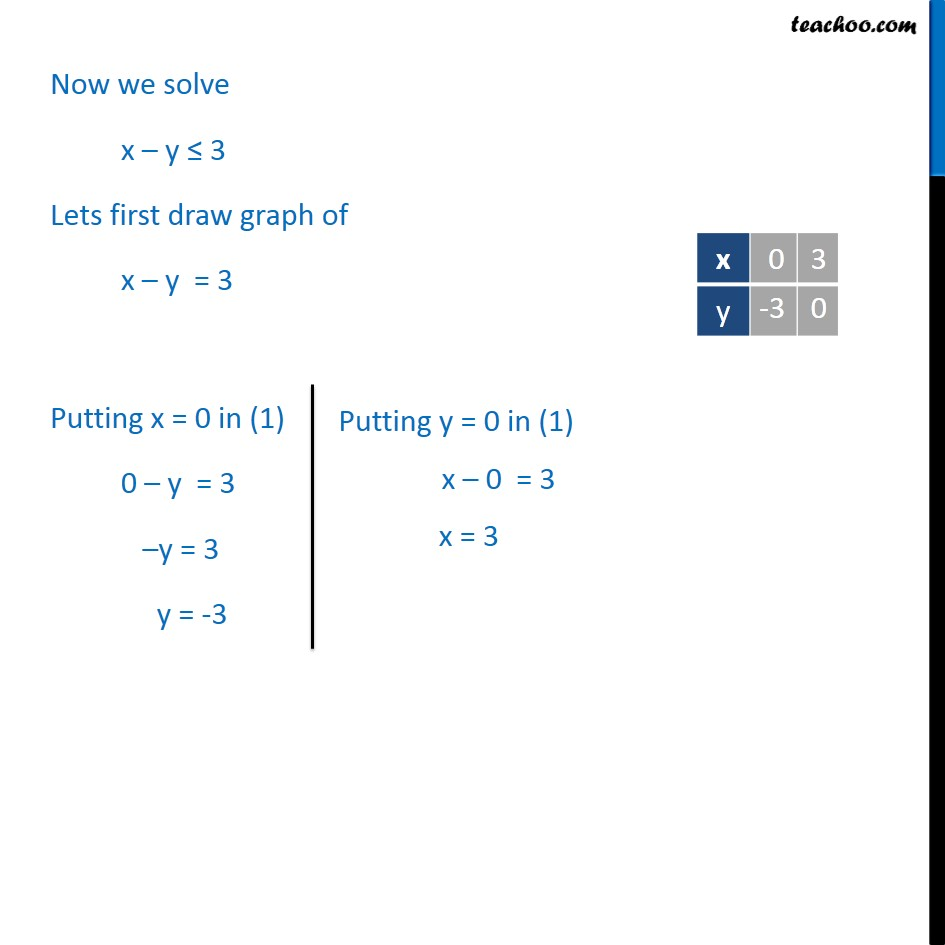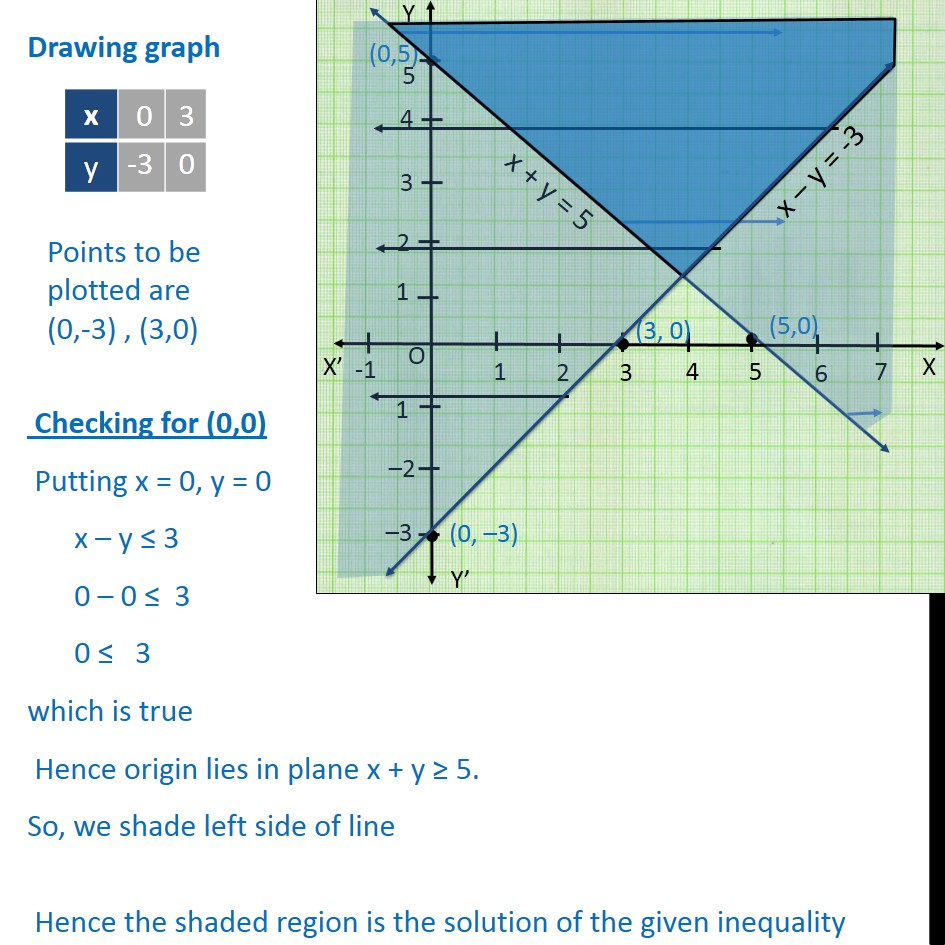Subscribe to our Youtube Channel - https://you.tube/teachoo

1. Chapter 6 Class 11 Linear Inequalities
2. Concept wise
3. Graph - 2 or more Equation

Transcript

Example 12 Solve the following system of linear inequalities graphically. x + y ≥ 5 , x – y ≤ 3 First we solve x + y ≥ 5 Lets first draw graph of x + y = 5 Drawing graph Checking for (0,0) Putting x = 0, y = 0 x + y ≥ 5 0 + 0 ≥ 5 0 ≥ 5 which is false Hence origin does not lie in plane x + y ≥ 5. So, we shade the right side of line Now we solve x – y ≤ 3 Lets first draw graph of x – y = 3 Drawing graph Checking for (0,0) Putting x = 0, y = 0 x – y ≤ 3 0 – 0 ≤ 3 0 ≤ 3 which is true Hence origin lies in plane x + y ≥ 5. So, we shade left side of line Hence the shaded region is the solution of the given inequality

Graph - 2 or more Equation